# Feedback Amplifier Design

This example shows the design of a noninverting feedback amplifier circuit using Control System Toolbox™. This design is built around the operational amplifier (op amp), a standard building block of electrical feedback circuits.

The example shows how a real electrical system can be designed, modeled, and analyzed using the tools provided by Control System Toolbox.

### Op Amp Description

The standard building block of electrical feedback circuits is the operational amplifier (op amp), a differential voltage amplifier designed to have extremely high dc gain, often in the range of 1e5 to 1e7.

The electrical symbol for the op amp is shown below.This example assumes the use of an uncompensated op amp with two poles (at frequencies w1,w2) and high dc gain (a0). Assuming this op amp is operated in its linear mode (not saturated), then its open-loop transfer function can be represented as a linear time-invariant (LTI) system, as shown above.

Though higher-order poles exist for a physical op amp, this example assumes that these poles lie in a frequency range where the magnitude is well below unity.

The open-loop transfer functions for this op amp is as follows.

`$a\left(s\right)=\frac{{a}_{0}}{\left(1+s/{\omega }_{1}\right)\left(1+s/{\omega }_{2}\right)}$`

For this example, assume the following transfer function parmaters.

`${a}_{0}=1e5$`

`${\omega }_{1}=1e4$`

`${\omega }_{2}=1e6$`

```a0 = 1e5; w1 = 1e4; w2 = 1e6;```

Create a transfer function model of this system using a Control System Toolbox LTI model.

First, define the Laplace variable `s` using the `tf` command. Then use `s` to construct the open-loop transfer function a.

```s = tf('s'); a = a0/(1+s/w1)/(1+s/w2)```
```a = 1e15 ---------------------- s^2 + 1.01e06 s + 1e10 Continuous-time transfer function. ```

View the frequency response of this system.

```h = bodeplot(a,'r'); setoptions(h, ... 'FreqUnits','rad/s', ... 'MagUnits','dB', ... 'PhaseUnits','deg',... 'YLimMode','Manual', ... 'YLim',{[0,110],[-180,0]});```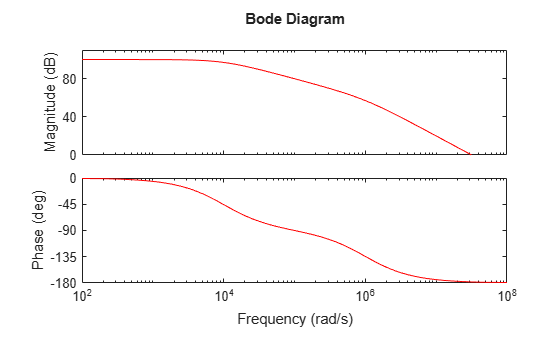View the normalized step response of `a` using the `stepplot` and `dcgain` commands.

```a_norm = a / dcgain(a); stepplot(a_norm,'r') title('Normalized Open-Loop Step Response') ylabel('Normalized Amplitude')```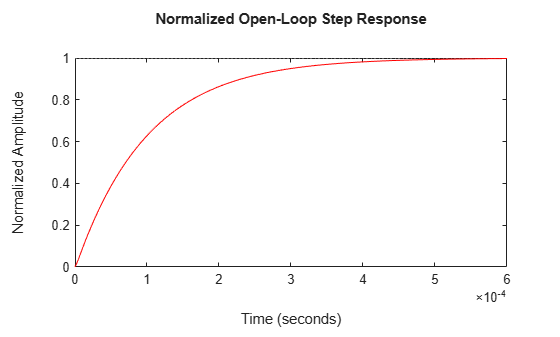Right-click on the plot and select "Characteristics -> Settling Time" to display the settling time. Hold the mouse over the settling time marker to reveal the exact value of the settling time.

### Feedback Amplifier

Now add a resistive feedback network and wire the system as a noninverting amplifier.This feedback network, `b`, is simply a voltage divider with input `Vo` and output `Vn`. Solving for the ratio `Vn`/`Vo` yields the transfer function for `b`.

``` b = Vn / Vo = R1 / (R1 + R2) ```

The block diagram representation of the system is shown below.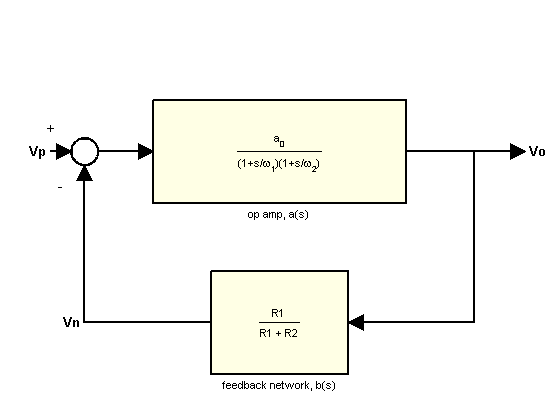Solving for the ratio Vo/Vp yields the closed-loop gain, `A`.

``` A = Vo / Vp = a / (1 + ab) ```

If the product od `a` and `b` is sufficiently large (>>1), then `A` can be approximated as:

``` A = 1 / b ```

Assume that you need to design an amplifier of dc gain (Vo/Vp) 10 and that `R1` is fixed at 10 kOhm. Solve for `R2`.

```A0 = 10; b = 1 / A0; % approximation for ab>>1 R1 = 10000; R2 = R1 * (1/b - 1)```
```R2 = 90000 ```

Construct the closed-loop system using the `feedback` command:

`A = feedback(a,b);`

Plot the frequency responses of `a` and `A` together using the `bodemag` command:

```bodemag(a,'r',A,'b'); legend('Open-Loop Gain (a)','Closed-Loop Gain (A)') ylim([0,110]); % Annotations opampdemo_annotate(1)```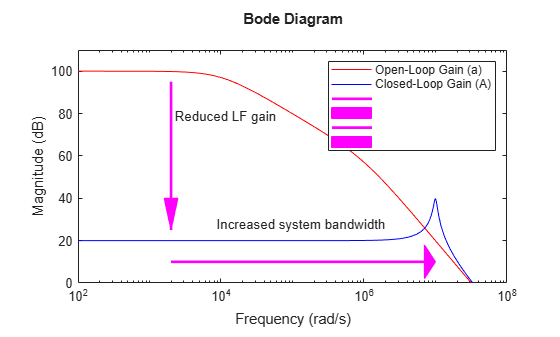The use of negative feedback to reduce the low-frequency (LF) gain has led to a corresponding increase in the system bandwidth (defined as the frequency where the gain drops 3 dB below its maximum value).

This gain versus bandwidth tradeoff is a powerful tool in the design of feedback amplifier circuits.

Since the gain is now dominated by the feedback network, a useful relationship to consider is the sensitivity of this gain to variation in the op amp's natural (open-loop) gain.

Before deriving the system sensitivity, however, it is useful to define the loop gain, `L`, which is the total gain a signal experiences traveling around the loop:

`L = a * b;`

You will use this quantity to evaluate the system sensitivity and stability margins.

The system sensitivity, `S`, represents the sensitivity of `A` to variation in `a`.

`$S=\frac{\delta A/A}{\delta a/a}=\frac{1}{1+a\left(s\right)b\left(s\right)}=\frac{1}{1+L\left(s\right)}$`

The inverse relationship between `S` and `L` reveals another benefit of negative feedback, gain desensitivity.

`S = 1 / (1 + L);`

`S` has the same form as the feedback equation and, therefore, can be constructed using the more-robust `feedback` command:

`S = feedback(1,L);`

The magnitudes of `S` and `A` may be plotted together using the `bodemag` command:

```bodemag(A,'b',S,'g') legend('Closed-Loop Gain(A)','System Sensitivity(S)', ... 'Location','SouthEast')```The very small low-frequency sensitivity (about -80 dB) indicates a design whose closed-loop gain suffers minimally from open-loop gain variation. Such variation in `a` is common due to manufacturing variability, temperature change, and so on.

You can check the step response of `A` using the `stepplot` command:

```stepplot(A) % Annotation opampdemo_annotate(2)```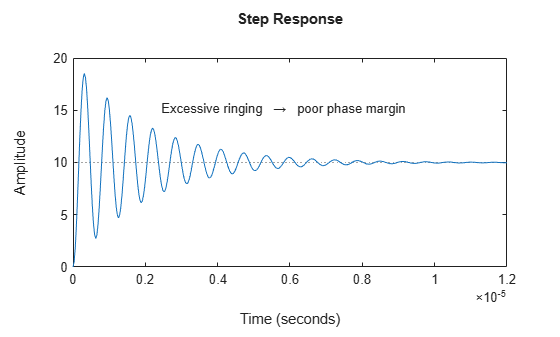The use of feedback has greatly reduced the settling time (by about 98%). However, the step response now displays a large amount of ringing, indicating poor stability margin.

You can analyze the stability margin by plotting the loop gain, `L`, with the `margin` command:

`margin(L)`The resulting plot indicates a phase margin of less than 6 degrees. You will need to compensate this amplifier in order to raise the phase margin to an acceptable level (generally 45 deg or more), thus reducing excessive overshoot and ringing.

A commonly used method of compensation in this type of circuit is "feedback lead compensation". This technique modifies b(s) by adding a capacitor, `C`, in parallel with the feedback resistor, `R2`.The capacitor value is chosen so as to introduce a phase lead to `b` near the crossover frequency, thus increasing the amplifier phase margin.

The new feedback transfer function is shown below.You can approximate a value for `C` by placing the zero of `b` at the 0 dB crossover frequency of `L`:

```[Gm,Pm,Wcg,Wcp] = margin(L); C = 1/(R2*Wcp)```
```C = 1.1139e-12 ```

To study the effect of `C` on the amplifier response, create an LTI model array of `b` for several values of `C` around your initial guess: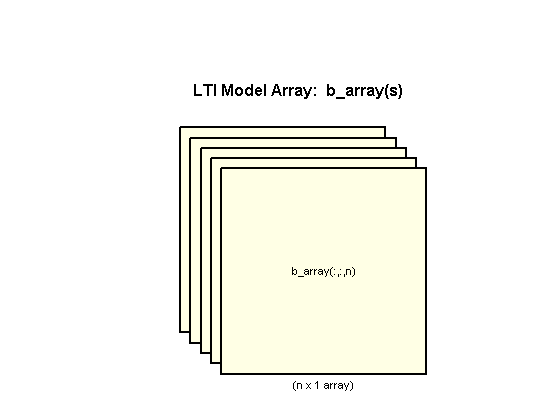```K = R1/(R1+R2); C = [1:.2:3]*1e-12; for n = 1:length(C) b_array(:,:,n) = tf([K*R2*C(n) K],[K*R2*C(n) 1]); end```

You can create LTI arrays for `A` and `L`.

```A_array = feedback(a,b_array); L_array = a*b_array;```

You can plot the step response of all models in the LTI array, `A_array`, together with `A` using the `stepplot` command.

```stepplot(A,'b:',A_array,'b',[0:.005:1]*1.5e-6) title('Closed-Loop Step Response (Compensated)') % Plot Annotations opampdemo_annotate(3)```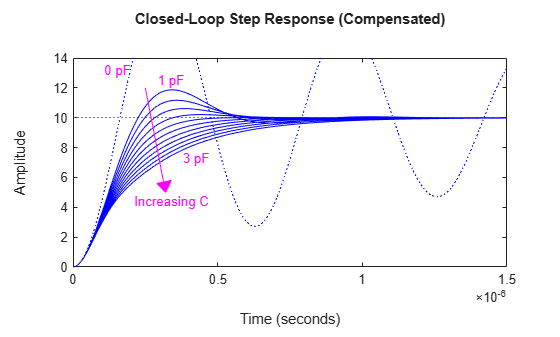The phase margins for our loop gain array,` L_array`, are found using the` margin` command.

`[Gm,Pm,Wcg,Wcp] = margin(L_array);`

The phase margins may now be plotted as a function of C.

```plot(C*1e12,Pm,'g') ax = gca; xlim([0.8 3.6]) ylim([45 60]) ax.Box = 'on'; xlabel('Compensation Capacitor, C (pF)') ylabel('Phase Margin (deg)') % Plot Annotations opampdemo_annotate(4)```A maximum phase margin of 58 deg is obtained when `C` = 2 pF (2e-12).

The model corresponding to `C` = 2 pF is the sixth model in the LTI array, `b_array`. You can plot the step response of the closed-loop system for this model by selecting index 6 of the LTI array `A_array`.

```A_comp = A_array(:,:,6); stepplot(A,'b:',A_comp,'b') legend('Uncompensated (0 pF)','Compensated (2 pF)')```The settling time has been further reduced (by an additional 85%).

You can overlay the frequency-response of all three models (open-loop, closed-loop, compensated closed-loop) using the `bode` command:

```bodeplot(a,'r',A,'b:',A_comp,'b') legend('a(s)','A(s)','A\_comp(s)')```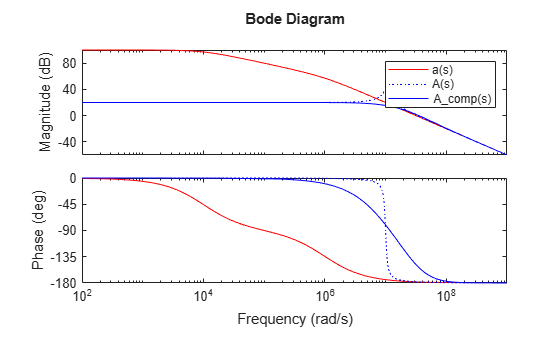The addition of the compensation capacitor eliminates peaking in the closed-loop gain and also greatly extended the phase margin.

### Summary

A brief summary of the choice of component values in the design of this non-inverting feedback amplifier circuit:

• Final component values: R1 = 10 kOhm, R2 = 90 kOhm, C = 2 pF.

• A resistive feedback network (R1,R2) was selected to yield a broadband amplifier gain of 10 (20 dB).

• Feedback lead compensation was used to tune the loop gain near the crossover frequency. The value for the compensation capacitor, C, was optimized to provide a maximum phase margin of about 58 degrees.